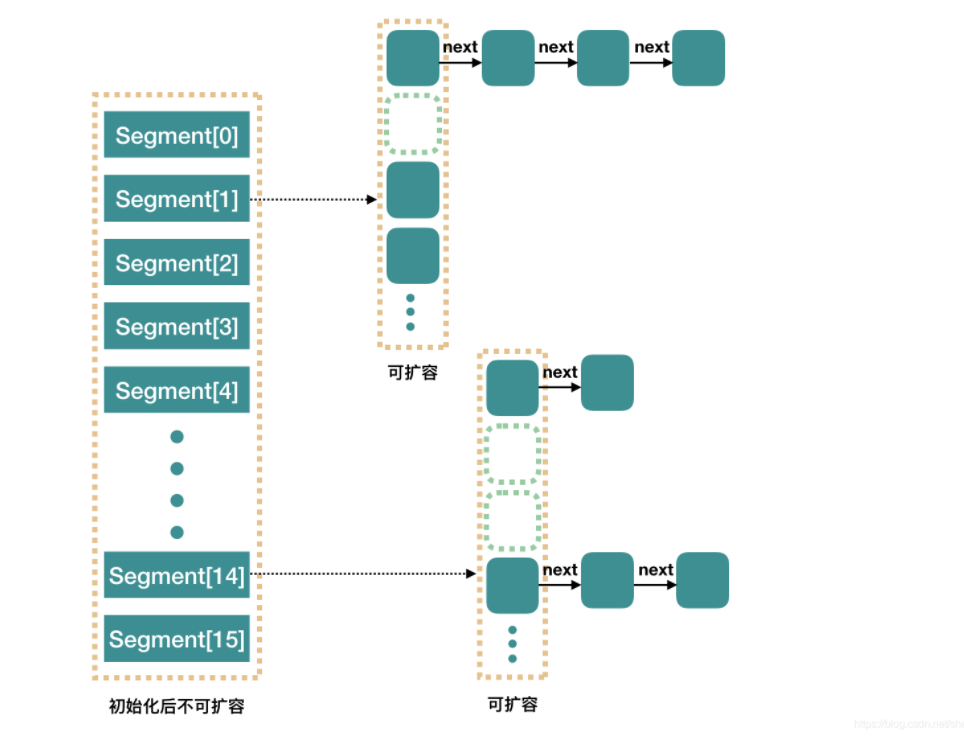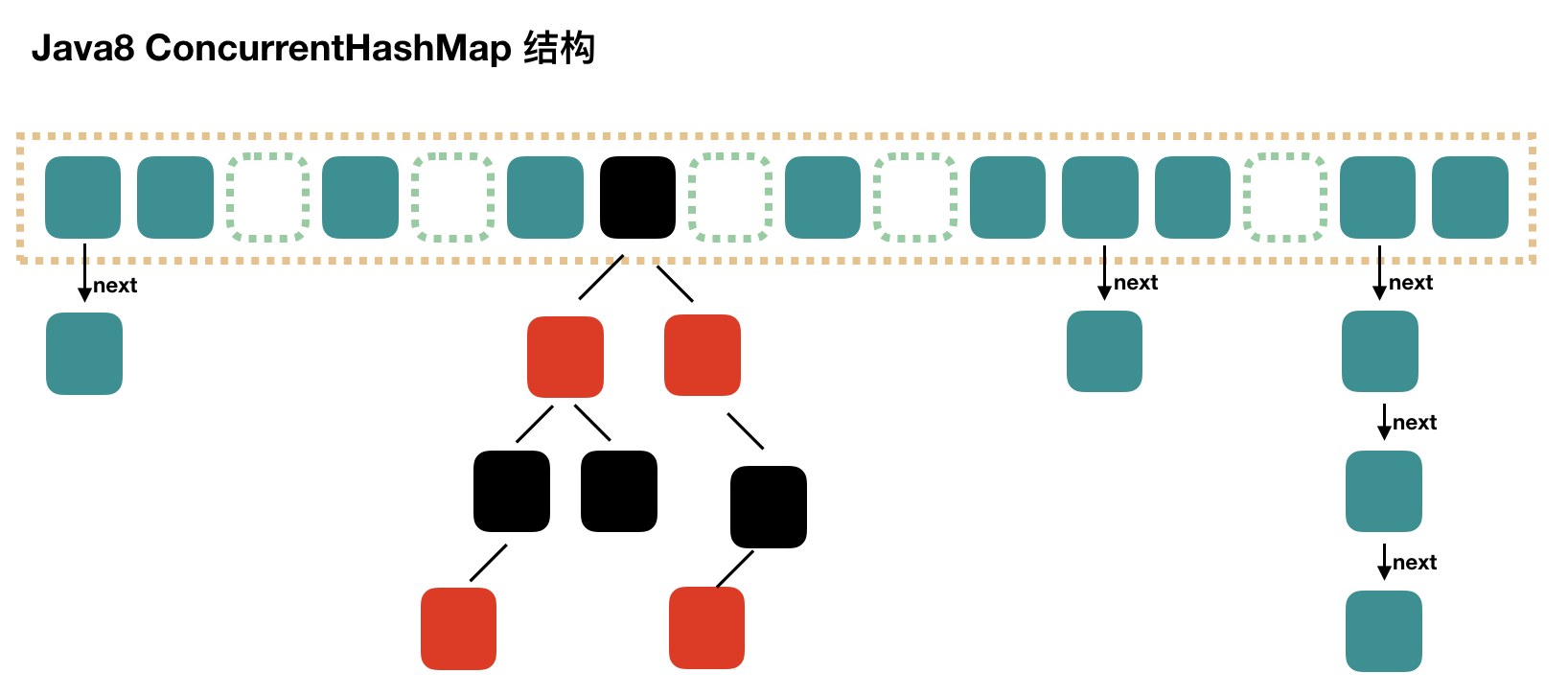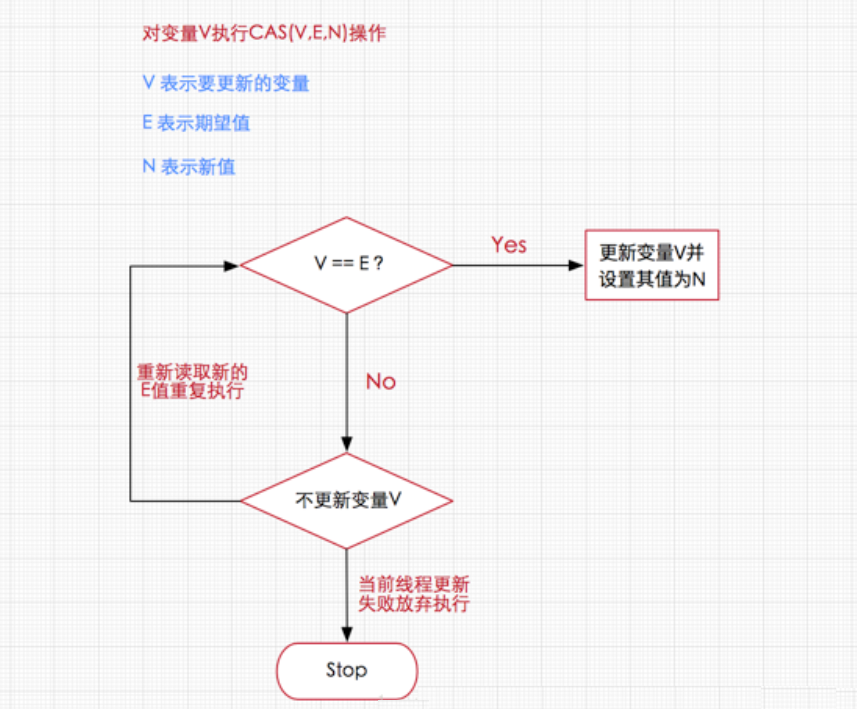﻿ 翻了ConcurrentHashMap1.7 和1.8的源碼，我總結了它們的主要區別。_ZenDei技術網路在線

# 翻了ConcurrentHashMap1.7 和1.8的源碼，我總結了它們的主要區別。ConcurrentHashMap 思考：HashTable是線程安全的，為什麼不推薦使用？ HashTable是一個線程安全的類，它使用synchronized來鎖住整張Hash表來實現線程安全，即每次鎖住整張表讓線程獨占，相當於所有線程進行讀寫時都去競爭一把鎖，導致效率非常低下。 1 Concu ...

## ConcurrentHashMap

HashTable是一個線程安全的類，它使用synchronized來鎖住整張Hash表來實現線程安全，即每次鎖住整張表讓線程獨占，相當於所有線程進行讀寫時都去競爭一把鎖，導致效率非常低下。

### 1 ConcurrentHashMap 1.7

Segment(分段鎖)-減少鎖的粒度

ConcurrentHashMap中的分段鎖稱為Segment，它即類似於HashMap的結構，即內部擁有一個Entry數組，數組中的每個元素又是一個鏈表,同時又是一個ReentrantLock（Segment繼承了ReentrantLock）。

#### 1.存儲結構

Java 7 版本 ConcurrentHashMap 的存儲結構如圖：ConcurrnetHashMap 由很多個 Segment 組合，而每一個 Segment 是一個類似於 HashMap 的結構，所以每一個 HashMap 的內部可以進行擴容。但是 Segment 的個數一旦初始化就不能改變，預設 Segment 的個數是 16 個，所以可以認為 ConcurrentHashMap 預設支持最多 16 個線程併發。

#### 2. 初始化

``````    /**
* Creates a new, empty map with a default initial capacity (16),
* load factor (0.75) and concurrencyLevel (16).
*/
public ConcurrentHashMap() {
}
``````

``````    /**
* 預設初始化容量,這個容量指的是Segment 的大小
*/
static final int DEFAULT_INITIAL_CAPACITY = 16;

/**
* 預設負載因數
*/
static final float DEFAULT_LOAD_FACTOR = 0.75f;

/**
* 預設併發級別，併發級別指的是Segment桶的個數，預設是16個併發大小
*/
static final int DEFAULT_CONCURRENCY_LEVEL = 16;

Segment下麵entryset數組的大小是用DEFAULT_INITIAL_CAPACITY/DEFAULT_CONCURRENCY_LEVEL求出來的。
``````

``````@SuppressWarnings("unchecked")
public ConcurrentHashMap(int initialCapacity,float loadFactor, int concurrencyLevel) {
// 參數校驗
if (!(loadFactor > 0) || initialCapacity < 0 || concurrencyLevel <= 0)
throw new IllegalArgumentException();
// 校驗併發級別大小，大於 1<<16，重置為 65536
if (concurrencyLevel > MAX_SEGMENTS)
concurrencyLevel = MAX_SEGMENTS;
// Find power-of-two sizes best matching arguments
// 2的多少次方
int sshift = 0;//控制segment數組的大小
int ssize = 1;
// 這個迴圈可以找到 concurrencyLevel 之上最近的 2的次方值
while (ssize < concurrencyLevel) {
++sshift;//代表ssize左移的次數
ssize <<= 1;
}
// 記錄段偏移量
this.segmentShift = 32 - sshift;
// 記錄段掩碼
// 設置容量   判斷初始容量是否超過允許的最大容量
if (initialCapacity > MAXIMUM_CAPACITY)
initialCapacity = MAXIMUM_CAPACITY;
// c = 容量 / ssize ，預設 16 / 16 = 1，這裡是計算每個 Segment 中的類似於 HashMap 的容量
//求entrySet數組的大小，這個地方需要保證entrySet數組的大小至少可以存儲下initialCapacity的容量，假設initialCapacity為33，ssize為16，那麼c=2,所以if語句是true，那麼c=3,MIN_SEGMENT_TABLE_CAPACITY初始值是2，所以if語句成立，那麼cap=4，所以每一個segment的容量初始為4，segment為16，16*4>33成立，entrySet數組的大小也需要是2的冪次方
int c = initialCapacity / ssize;
if (c * ssize < initialCapacity)
++c;
int cap = MIN_SEGMENT_TABLE_CAPACITY;
//Segment 中的類似於 HashMap 的容量至少是2或者2的倍數
while (cap < c)
cap <<= 1;
// create segments and segments
// 創建 Segment 數組，設置 segments
(HashEntry<K,V>[])new HashEntry[cap]);
Segment<K,V>[] ss = (Segment<K,V>[])new Segment[ssize];
UNSAFE.putOrderedObject(ss, SBASE, s0); // ordered write of segments
this.segments = ss;
}
``````

1. 必要參數校驗。
2. 校驗併發級別 concurrencyLevel 大小，如果大於最大值，重置為最大值。無參構造預設值是 16.
3. 尋找併發級別 concurrencyLevel 之上最近的 2 的冪次方值，作為初始化容量大小，預設是 16
4. 記錄 segmentShift 偏移量，這個值為【容量 = 2 的N次方】中的 N，在後面 Put 時計算位置時會用到。預設是 32 - sshift = 28.
5. 記錄 segmentMask，預設是 ssize - 1 = 16 -1 = 15.
6. 初始化 segments預設大小為 2負載因數 0.75擴容閥值是 2*0.75=1.5，插入第二個值時才會進行擴容。
1. 計算segment數組容量的大小。
2. 計算entrySet數組的大小。
3. 初始化segment數組，其中生成一個s0對象放在數組的第0個位置
4. 為什麼首先需要一個s0存儲到數組的第一個位置？

1. Segment數組的長度第一次已經確定，以後不會在改變，擴容是局部擴容，只對setrySet數組的容量進行擴容。

#### 3. put

``````/**
* Maps the specified key to the specified value in this table.
* Neither the key nor the value can be null.
*
* <p> The value can be retrieved by calling the <tt>get</tt> method
* with a key that is equal to the original key.
*
* @param key key with which the specified value is to be associated
* @param value value to be associated with the specified key
* @return the previous value associated with <tt>key</tt>, or
*         <tt>null</tt> if there was no mapping for <tt>key</tt>
* @throws NullPointerException if the specified key or value is null
*/
public V put(K key, V value) {
Segment<K,V> s;
if (value == null)
throw new NullPointerException();
int hash = hash(key);
// hash 值無符號右移 28位（初始化時獲得），然後與 segmentMask=15 做與運算

// this.segmentMask = ssize - 1;
//對hash值進行右移segmentShift位，計算元素對應segment中數組下表的位置
int j = (hash >>> segmentShift) & segmentMask;
//使用unsafe對象獲取數組中第j個位置的值，後面加上的是偏移量
if ((s = (Segment<K,V>)UNSAFE.getObject          // nonvolatile; recheck
(segments, (j << SSHIFT) + SBASE)) == null) //  in ensureSegment
// 如果查找到的 Segment 為空，初始化
s = ensureSegment(j);
//插入segment對象
return s.put(key, hash, value, false);
}

/**
* Returns the segment for the given index, creating it and
* recording in segment table (via CAS) if not already present.
*
* @param k the index
* @return the segment
*/
@SuppressWarnings("unchecked")
private Segment<K,V> ensureSegment(int k) {
final Segment<K,V>[] ss = this.segments;
long u = (k << SSHIFT) + SBASE; // raw offset
Segment<K,V> seg;
// 判斷 u 位置的 Segment 是否為null
if ((seg = (Segment<K,V>)UNSAFE.getObjectVolatile(ss, u)) == null) {
Segment<K,V> proto = ss; // use segment 0 as prototype
// 獲取0號 segment 里的 HashEntry<K,V> 初始化長度
int cap = proto.table.length;
// 獲取0號 segment 里的 hash 表裡的擴容負載因數，所有的 segment 的 loadFactor 是相同的
// 計算擴容閥值
int threshold = (int)(cap * lf);
// 創建一個 cap 容量的 HashEntry 數組
HashEntry<K,V>[] tab = (HashEntry<K,V>[])new HashEntry[cap];
if ((seg = (Segment<K,V>)UNSAFE.getObjectVolatile(ss, u)) == null) { // recheck
// 再次檢查 u 位置的 Segment 是否為null，因為這時可能有其他線程進行了操作
Segment<K,V> s = new Segment<K,V>(lf, threshold, tab);
// 自旋檢查 u 位置的 Segment 是否為null
while ((seg = (Segment<K,V>)UNSAFE.getObjectVolatile(ss, u))
== null) {
// 使用CAS 賦值，只會成功一次
if (UNSAFE.compareAndSwapObject(ss, u, null, seg = s))
break;
}
}
}
return seg;
}
``````

1. 計算要 put 的 key 的位置，獲取指定位置的 Segment。

2. 如果指定位置的 Segment 為空，則初始化這個 Segment.

初始化 Segment 流程：

1. 檢查計算得到的位置的 Segment 是否為null.
2. 為 null 繼續初始化，使用 Segment 的容量和負載因數創建一個 HashEntry 數組。
3. 再次檢查計算得到的指定位置的 Segment 是否為null.
4. 使用創建的 HashEntry 數組初始化這個 Segment.
5. 自旋判斷計算得到的指定位置的 Segment 是否為null，使用 CAS 在這個位置賦值為 Segment.
3. Segment.put 插入 key,value 值。

``````final V put(K key, int hash, V value, boolean onlyIfAbsent) {
// 獲取 ReentrantLock 獨占鎖，獲取不到，scanAndLockForPut 獲取。
HashEntry<K,V> node = tryLock() ? null : scanAndLockForPut(key, hash, value);
V oldValue;
try {
HashEntry<K,V>[] tab = table;
// 計算要put的數據位置
int index = (tab.length - 1) & hash;
// CAS 獲取 index 坐標的值
HashEntry<K,V> first = entryAt(tab, index);
for (HashEntry<K,V> e = first;;) {
if (e != null) {
// 檢查是否 key 已經存在，如果存在，則遍歷鏈表尋找位置，找到後替換 value
K k;
if ((k = e.key) == key ||
(e.hash == hash && key.equals(k))) {
oldValue = e.value;
if (!onlyIfAbsent) {
e.value = value;
++modCount;
}
break;
}
e = e.next;
}
else {
// first 有值沒說明 index 位置已經有值了，有衝突，鏈表頭插法。
if (node != null)
node.setNext(first);
else
node = new HashEntry<K,V>(hash, key, value, first);
int c = count + 1;
// 容量大於擴容閥值，小於最大容量，進行擴容
if (c > threshold && tab.length < MAXIMUM_CAPACITY)
rehash(node);
else
// index 位置賦值 node，node 可能是一個元素，也可能是一個鏈表的表頭
setEntryAt(tab, index, node);
++modCount;
count = c;
oldValue = null;
break;
}
}
} finally {
unlock();
}
return oldValue;
}
``````

1. tryLock() 獲取鎖，獲取不到使用 `scanAndLockForPut` 方法繼續獲取。

2. 計算 put 的數據要放入的 index 位置，然後獲取這個位置上的 HashEntry 。

3. 遍歷 put 新元素，為什麼要遍歷？因為這裡獲取的 HashEntry 可能是一個空元素，也可能是鏈表已存在，所以要區別對待。

如果這個位置上的 HashEntry 不存在

1. 如果當前容量大於擴容閥值，小於最大容量，進行擴容
2. 直接頭插法插入。

如果這個位置上的 HashEntry 存在

1. 判斷鏈表當前元素 Key 和 hash 值是否和要 put 的 key 和 hash 值一致。一致則替換值
2. 不一致，獲取鏈表下一個節點，直到發現相同進行值替換，或者鏈表表裡完畢沒有相同的。
1. 如果當前容量大於擴容閥值，小於最大容量，進行擴容
2. 直接鏈表頭插法插入。
4. 如果要插入的位置之前已經存在，替換後返回舊值，否則返回 null.

``````private HashEntry<K,V> scanAndLockForPut(K key, int hash, V value) {
HashEntry<K,V> first = entryForHash(this, hash);
HashEntry<K,V> e = first;
HashEntry<K,V> node = null;
int retries = -1; // negative while locating node
// 自旋獲取鎖
while (!tryLock()) {
HashEntry<K,V> f; // to recheck first below
if (retries < 0) {
if (e == null) {
if (node == null) // speculatively create node
node = new HashEntry<K,V>(hash, key, value, null);
retries = 0;
}
else if (key.equals(e.key))
retries = 0;
else
e = e.next;
}
else if (++retries > MAX_SCAN_RETRIES) {
// 自旋達到指定次數後，阻塞等到只到獲取到鎖
lock();
break;
}
else if ((retries & 1) == 0 &&
(f = entryForHash(this, hash)) != first) {
e = first = f; // re-traverse if entry changed
retries = -1;
}
}
return node;
}

``````

#### 4. 擴容 rehash

ConcurrentHashMap 的擴容只會擴容到原來的兩倍。老數組裡的數據移動到新的數組時，位置要麼不變，要麼變為 index+ oldSize，參數里的 node 會在擴容之後使用鏈表頭插法插入到指定位置。

``````private void rehash(HashEntry<K,V> node) {
HashEntry<K,V>[] oldTable = table;
// 老容量
int oldCapacity = oldTable.length;
// 新容量，擴大兩倍
int newCapacity = oldCapacity << 1;
// 新的擴容閥值
// 創建新的數組
HashEntry<K,V>[] newTable = (HashEntry<K,V>[]) new HashEntry[newCapacity];
// 新的掩碼，預設2擴容後是4，-1是3，二進位就是11。
int sizeMask = newCapacity - 1;
for (int i = 0; i < oldCapacity ; i++) {
// 遍歷老數組
HashEntry<K,V> e = oldTable[i];
if (e != null) {
HashEntry<K,V> next = e.next;
// 計算新的位置，新的位置只可能是不便或者是老的位置+老的容量。
int idx = e.hash & sizeMask;
if (next == null)   //  Single node on list
// 如果當前位置還不是鏈表，只是一個元素，直接賦值
newTable[idx] = e;
else { // Reuse consecutive sequence at same slot
// 如果是鏈表了
HashEntry<K,V> lastRun = e;
int lastIdx = idx;
// 新的位置只可能是不便或者是老的位置+老的容量。
// 遍歷結束後，lastRun 後面的元素位置都是相同的
for (HashEntry<K,V> last = next; last != null; last = last.next) {
int k = last.hash & sizeMask;
if (k != lastIdx) {
lastIdx = k;
lastRun = last;
}
}
// ，lastRun 後面的元素位置都是相同的，直接作為鏈表賦值到新位置。
newTable[lastIdx] = lastRun;
// Clone remaining nodes
for (HashEntry<K,V> p = e; p != lastRun; p = p.next) {
// 遍歷剩餘元素，頭插法到指定 k 位置。
V v = p.value;
int h = p.hash;
int k = h & sizeMask;
HashEntry<K,V> n = newTable[k];
newTable[k] = new HashEntry<K,V>(h, p.key, v, n);
}
}
}
}
// 頭插法插入新的節點
node.setNext(newTable[nodeIndex]);
newTable[nodeIndex] = node;
table = newTable;
}
``````

#### 5. get

1. 計算得到 key 的存放位置。
2. 遍歷指定位置查找相同 key 的 value 值。
``````public V get(Object key) {
Segment<K,V> s; // manually integrate access methods to reduce overhead
HashEntry<K,V>[] tab;
int h = hash(key);
long u = (((h >>> segmentShift) & segmentMask) << SSHIFT) + SBASE;
// 計算得到 key 的存放位置
if ((s = (Segment<K,V>)UNSAFE.getObjectVolatile(segments, u)) != null &&
(tab = s.table) != null) {
for (HashEntry<K,V> e = (HashEntry<K,V>) UNSAFE.getObjectVolatile
(tab, ((long)(((tab.length - 1) & h)) << TSHIFT) + TBASE);
e != null; e = e.next) {
// 如果是鏈表，遍歷查找到相同 key 的 value。
K k;
if ((k = e.key) == key || (e.hash == h && key.equals(k)))
return e.value;
}
}
return null;
}
``````

### 2 ConcurrentHashMap 1.8

#### 1. 存儲結構CAS（Compare-and-Swap/Exchange），即比較並替換，是一種實現併發常用到的技術。

CAS核心演算法：執行函數：CAS(V，E，N)

``````V表示準備要被更新的變數 （記憶體的值）
E表示我們提供的 期望的值 （期望的原值）
N表示新值 ，準備更新V的值 （新值）
````````````   由於CAS操作屬於樂觀派，它總是認為自己能夠操作成功，所以操作失敗的線程將會再次發起操作，而不是被OS掛起。所以說，即使CAS操作沒有使用同步鎖，其它線程也能夠知道對共用變數的影響。

因為其它線程沒有被掛起，並且將會再次發起修改嘗試，所以無鎖操作即CAS操作天生免疫死鎖。

另外一點需要知道的是，CAS是系統原語，CAS操作是一條CPU的原子指令，所以不會有線程安全問題。
``````

ABA問題：E和E2對比相同是不能保證百分百保證，其他線程沒有在自己線程執行計算的過程里搶鎖成功過。有可能其他線程操作後新E值和舊E值一樣！

ABA問題解決：在E對象裡加個操作次數變數就行，每次判斷時對比兩個，E和操作次數就OK了，因為ABA問題中就算E相同操作次數也絕不相同

#### 2. 初始化 initTable

``````/**
* Initializes table, using the size recorded in sizeCtl.
*/
private final Node<K,V>[] initTable() {
Node<K,V>[] tab; int sc;
while ((tab = table) == null || tab.length == 0) {
//如果 sizeCtl < 0 ,說明另外的線程執行CAS 成功，正在進行初始化。
if ((sc = sizeCtl) < 0)
// 讓出 CPU 使用權
Thread.yield(); // lost initialization race; just spin
else if (U.compareAndSwapInt(this, SIZECTL, sc, -1)) {
try {
if ((tab = table) == null || tab.length == 0) {
int n = (sc > 0) ? sc : DEFAULT_CAPACITY;
@SuppressWarnings("unchecked")
Node<K,V>[] nt = (Node<K,V>[])new Node<?,?>[n];
table = tab = nt;
sc = n - (n >>> 2);
}
} finally {
sizeCtl = sc;
}
break;
}
}
return tab;
}
``````

1. -1 說明正在初始化
2. -N 說明有N-1個線程正在進行擴容
3. 表示 table 初始化大小，如果 table 沒有初始化
4. 表示 table 容量，如果 table　已經初始化。

#### 3. put

``````public V put(K key, V value) {
return putVal(key, value, false);
}

/** Implementation for put and putIfAbsent */
final V putVal(K key, V value, boolean onlyIfAbsent) {
// key 和 value 不能為空
if (key == null || value == null) throw new NullPointerException();
int binCount = 0;
for (Node<K,V>[] tab = table;;) {
// f = 目標位置元素
Node<K,V> f; int n, i, fh;// fh 後面存放目標位置的元素 hash 值
if (tab == null || (n = tab.length) == 0)
// 數組桶為空，初始化數組桶（自旋+CAS)
tab = initTable();
else if ((f = tabAt(tab, i = (n - 1) & hash)) == null) {
// 桶內為空，CAS 放入，不加鎖，成功了就直接 break 跳出
if (casTabAt(tab, i, null,new Node<K,V>(hash, key, value, null)))
break;  // no lock when adding to empty bin
}
else if ((fh = f.hash) == MOVED)
tab = helpTransfer(tab, f);
else {
V oldVal = null;
// 使用 synchronized 加鎖加入節點
synchronized (f) {
if (tabAt(tab, i) == f) {
// 說明是鏈表
if (fh >= 0) {
binCount = 1;
// 迴圈加入新的或者覆蓋節點
for (Node<K,V> e = f;; ++binCount) {
K ek;
if (e.hash == hash &&
((ek = e.key) == key ||
(ek != null && key.equals(ek)))) {
oldVal = e.val;
if (!onlyIfAbsent)
e.val = value;
break;
}
Node<K,V> pred = e;
if ((e = e.next) == null) {
pred.next = new Node<K,V>(hash, key,
value, null);
break;
}
}
}
else if (f instanceof TreeBin) {
// 紅黑樹
Node<K,V> p;
binCount = 2;
if ((p = ((TreeBin<K,V>)f).putTreeVal(hash, key,
value)) != null) {
oldVal = p.val;
if (!onlyIfAbsent)
p.val = value;
}
}
}
}
if (binCount != 0) {
if (binCount >= TREEIFY_THRESHOLD)
treeifyBin(tab, i);
if (oldVal != null)
return oldVal;
break;
}
}
}
return null;
}
``````
1. 根據 key 計算出 hashcode 。
2. 判斷是否需要進行初始化。
3. 即為當前 key 定位出的 Node，如果為空表示當前位置可以寫入數據，利用 CAS 嘗試寫入，失敗則自旋保證成功。
4. 如果當前位置的 `hashcode == MOVED == -1`,則需要進行擴容。
5. 如果都不滿足，則利用 synchronized 鎖寫入數據。
6. 如果數量大於 `TREEIFY_THRESHOLD` 則要轉換為紅黑樹。

#### 4. get

get 流程比較簡單，直接過一遍源碼。

``````public V get(Object key) {
Node<K,V>[] tab; Node<K,V> e, p; int n, eh; K ek;
// key 所在的 hash 位置
if ((tab = table) != null && (n = tab.length) > 0 &&
(e = tabAt(tab, (n - 1) & h)) != null) {
// 如果指定位置元素存在，頭結點hash值相同
if ((eh = e.hash) == h) {
if ((ek = e.key) == key || (ek != null && key.equals(ek)))
// key hash 值相等，key值相同，直接返回元素 value
return e.val;
}
else if (eh < 0)
// 頭結點hash值小於0，說明正在擴容或者是紅黑樹，find查找
return (p = e.find(h, key)) != null ? p.val : null;
while ((e = e.next) != null) {
// 是鏈表，遍歷查找
if (e.hash == h &&
((ek = e.key) == key || (ek != null && key.equals(ek))))
return e.val;
}
}
return null;
}
``````

1. 根據 hash 值計算位置。
2. 查找到指定位置，如果頭節點就是要找的，直接返回它的 value.
3. 如果頭節點 hash 值小於 0 ，說明正在擴容或者是紅黑樹，查找之。
4. 如果是鏈表，遍歷查找之。

### 3 總結

Java7 中 ConcurrentHashMap 使用的分段鎖，也就是每一個 Segment 上同時只有一個線程可以操作，每一個 Segment 都是一個類似 HashMap 數組的結構，它可以擴容，它的衝突會轉化為鏈表。但是 Segment 的個數一但初始化就不能改變。

Java8 中的 ConcurrentHashMap 使用的 Synchronized 鎖加 CAS 的機制。結構也由 Java7 中的 Segment 數組 + HashEntry 數組 + 鏈表 進化成了 Node 數組 + 鏈表 / 紅黑樹，Node 是類似於一個 HashEntry 的結構。它的衝突再達到一定大小時會轉化成紅黑樹，在衝突小於一定數量時又退回鏈表。• 如何將編寫的c語言程式打包成exe可執行文件呢？ 以前我們寫程式很多是在編輯器上，讓編輯起來編譯運行我們的程式。如果想將其打包成exe可執行文件該如何做？ 我這裡推薦使用codeBlocks的gcc命令，因為比較簡單。像vs這種編輯器就像是一把屠龍刀。功能過於複雜，需要註意的很多。如何適用codeB ...
• 《Go 精進之路》 讀書筆記。簡要記錄自己打五角星的部分，方便複習鞏固。目前看到p120 Go 語言遵從的設計哲學為組合 垂直組合：類型嵌入，快速讓一個類型復用其他類型已經實現的能力，實現功能的垂直擴展。 水平組合：介面實現鴨子類型。 變數名字中不要帶有類型信息 userSlice []*User ...
• 怎麼借鑒開源代碼來打造一些自身面對的問題解決方案？也許有一些Demo來進行回答演示或許更為貼近地氣些。這裡打算寫一些玩轉源碼為主題的文字來實踐的回答，最近在看P3C的一些源碼，那就從這開始吧。 ...
• 什麼是 Session 會話? 1、Session 就一個介面（HttpSession）。 2、Session 就是會話。它是用來維護一個客戶端和伺服器之間關聯的一種技術。 3、每個客戶端都有自己的一個 Session 會話。 4、Session 會話中，我們經常用來保存用戶登錄之後的信息。 如何創 ...
• Spring中AOP的底層原理就是動態代理模式，所以我們在這裡對代理模式進行學習。 一、代理模式 1.什麼是代理 代理，顧名思義，就是一個人代替另一個人去做他需要做的事情。代理是一種設計模式，具體實現就是一個類代替某個類去實現功能。 我們舉一個例子： 我要租房子，我可以找房東直接租房子。同樣我可以找 ...
• 特殊說明：第一章只包含了 初始化上下文，初始化監聽器列表，發佈springboot啟動事件 相關內容 其中一部分代碼 /** * Run the Spring application, creating and refreshing a new * {@link ApplicationContext ...
• 前言 在使用SpringBoot的時候經常需要對客戶端傳入的參數進行合法性的校驗，校驗的方法基本上都是使用SpringBoot提供的註解，有時候遇上註解不能滿足需求的時候還需要在業務邏輯上進行判斷。這樣根本就沒有實現解耦。 使用方法 項目maven引入 <dependency> <groupId>c ...
• 0.基本的數據類型： Number（數字） String（字元串） List（列表） Tuple（元組） Set（集合） Dictionary（字典） 1.基本的輸入輸出: input #輸入 print #輸出 age = 10; name = "小明"; print(age,type(age)) ...